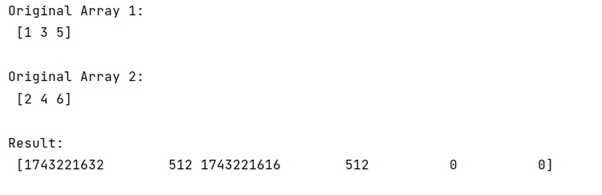# Interweaving two numpy arrays

Learn, how to interweave two numpy arrays in Python?
Submitted by Pranit Sharma, on January 08, 2023

NumPy is an abbreviated form of Numerical Python. It is used for different types of scientific operations in python. Numpy is a vast library in python which is used for almost every kind of scientific or mathematical operation. It is itself an array which is a collection of various methods and functions for processing the arrays.

Suppose that we are given two NumPy arrays and we need to interweave them efficiently so that one gets a third array.

For this purpose, we will simply use the size() method along with both the NumPy arrays and add the result and use this result inside the NumPy empty method and also define the data type.

Let us understand with the help of an example,

## Python code for interweaving two numpy arrays

```# Import numpy
import numpy as np

# Import pandas
import pandas as pd

# Creating two numpy arrays
arr = np.array([1,3,5])
arr2 = np.array([2,4,6])

# Display original arrays
print("Original Array 1:\n",arr,"\n")
print("Original Array 2:\n",arr2,"\n")

# Interweaving the arrays
res = np.empty((arr.size + arr2.size,), dtype=arr.dtype)

# Display result
print("Result:\n",res,"\n")
```

Output: